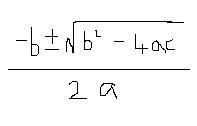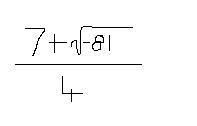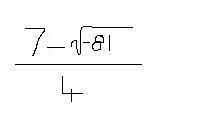Get your math homework solved here. - Printable Version +- School Survival Forums (http://forums.school-survival.net) +-- Forum: Learning, Youth Rights and School Survival (/forumdisplay.php?fid=3) +--- Forum: Homework Help & Answers (/forumdisplay.php?fid=30) +--- Thread: Get your math homework solved here. (/showthread.php?tid=12967) Pages: 1 2 3 Get your math homework solved here. - Michio-kun - 08-22-2008 10:57 AM Plz post lawl. Math is my strong point so ask away plz. I'll definitely answer. Also sorry but I can't do geometry. I can do anything up to calculus (and including calculus) except geometry. :( Edit: If you want personal help, I won't be coming on this forum anymore (hopefully), so if you want my help, you'll either have to email me (use the PM system to do this) or contact me (oneiromancy) at freemathhelp.com/forum. By the way, I'm also Gayarou here. Re: I'm doing everyone's math homework. - youvebeenthunderstruck - 08-22-2008 11:05 AM Two trains leave Harbor Station at 8:45 AM travelling west. After a while, the tracks seperate, Train A going north and Train B going south, each travelling at 45 mph. After 25 minutes, Train B accelerates to 60 mph. After an hour, Train A accelerates to 75 mph. Assuming both trains return to the station after 3 hours, what flavor is the blueberry pie? Re: I'm doing everyone's math homework. - Michio-kun - 08-22-2008 11:05 AM I lolled. Re: I'm doing everyone's math homework. - magikarp - 08-22-2008 11:08 AMHalp! (Find x. Also, I forgot to write in the cm part. But I don't think that's too important.) My math teacher is so mean. D: Re: I'm doing everyone's math homework. - youvebeenthunderstruck - 08-22-2008 11:09 AM fist: 5. Re: I'm doing everyone's math homework. - Specter - 08-22-2008 11:22 AM youvebeenthunderstruck Wrote:fist: 5. <.< >.> Stupid question, but what theory did you use ? Pythagorean theorem ? EDIT: ohhh or maybe the Cosine, Sine, Tangent Stuff ? Re: I'm doing everyone's math homework. - Bob Dole - 08-22-2008 11:24 AM Shadow: It's a Pythagorean triple. Re: I'm doing everyone's math homework. - Specter - 08-22-2008 11:27 AM ohThnx Re: I'm doing everyone's math homework. - Michio-kun - 08-22-2008 11:33 AM http://en.wikipedia.org/wiki/Pythagorean_triple 3^2 + 4^2 = x^2 9 + 16 = x^2 25 = x^2 So, take square roots of both sides and you get |x| = 5 or -5 Technically when you take the square root of even powers (in this case x^2), you get an absolute value, but in this case we can remove that absolute value because you can't have negative length, i.e. a side of that triangle you posted have -5 units length, this doesn't make any sense. so x = 5 Just for further explanation. The reason we had x = +5 or -5 when we took the square root of x^2 = 25 is because (-5)^2 = 25 but (5)^2 = 25 also. So -5 and 5 are both solutions to the equation x^2 = 25 . By "solution to the equation" I mean, what can we plug into the variable to create an equality (and thus a true statement)? Re: I'm doing everyone's math homework. - chibichibibear - 08-22-2008 02:29 PM Sweet! Are you serious? I fucking hate math(sorry...my last class kind a made me hate it when I realized I've used only up to basic algebra outside of school), and for some reason I'm going into pre-calculus.I'm pretty sure my next teacher does that packet HW method, where you turn in the weeks worth on Friday. I'll probably ask you to do like, 2 or 3 a week. So thank you so much in advance!(although I'll probably fail the tests... eh, I can pay a bit of attention in class) Although I feel really bad considering cheating like this... oh well, I'll get over it in like 5 minutes. So come Sep. 3rd, expect a lot of math. Re: I'm doing everyone's math homework. - Dark Soul X - 08-23-2008 04:26 AM I'll come back once school starts back up for me. Re: I'm doing everyone's math homework. - Trar - 08-29-2008 12:49 AM School starts Sept. 2 for me, so i'll post with any HW I have. But, the last grade I had required me to show all the work I had to do to get the answers, and if that's any indication of what to expect THIS year, i'm afraid you can't help me. Re: I'm doing everyone's math homework. - Gobinu - 08-29-2008 05:59 AM Man rly nice from yur side but tbh one want to know it or not. It is rly easy for a teacher to see that he konows nothing (u won't be with him in a classroom) I'd rather learn myself how to cheat at school (now i can so..) besides math is not so bad at least it is more or less clear , on polish (or other mother lenguagge lessonse) somtimes "2+2=5". Re: I'm doing everyone's math homework. - Slaughter - 08-29-2008 07:28 AM Last question of a math homework, worth 4,5 points, VERY IMPORTANT: With the numerical digits 0, 1, 2, 3, 4 and 5, determinate: a) How many numbers with three numerical digits can be made with those numbers? b) How many even numbers with three numerical digits can be made? c) How many odd numbers with three numerical digits can be made? d)How many numbers with two numerical digits can be made? If you can do so, please anwser until 7:00 AM in -3 GMT. I don't want to press you, but It's really important, like, life-deciding important. And yes, I'm a stupid dumbass. I really need to find a way to learn math, someone knows if there's such a way in the internet? Re: I'm doing everyone's math homework. - fragile-esteem - 09-02-2008 05:16 AM OK so, It's quadratic equations using a formula, and I'm stuck. you know the formula:Well I applied it to: 2x(squared)-7x+4 = 0 and then simplified and got:ORbut how does it work with a negative in the square route? my calculator keeps saying MATH ERROR! Re: I'm doing everyone's math homework. - Happy Camper - 09-02-2008 05:56 AM 2x^2 - 7x + 4 a = 2 ; b = -7 ; c = 4 7 +/- sqrt(49 - 4*2*4) 2*2 7 +/- sqrt(17) 4 I'm willing to bet you didn't put the -7 in parentheses when you squared it. That would cause you to come out with a negative 49 and a negative 32. When you square negative numbers, parenthesize the negative number. Or better yet, just plug it in as a positive number because you know it'll come out positive (if it's an even power). Re: I'm doing everyone's math homework. (old) - magikarp - 09-09-2008 03:11 AM IfThen evaluate:(I don't really need an answer for this, though. I can just skip it if no one knows.) Re: I'm doing everyone's math homework. (old) - Michio-kun - 09-09-2008 09:33 AM The above limit holds true when f(x) = sin x. => lim(x-->0) (sin (x) / x) = 1 => lim(x-->0) (sin (x)) = 0 For the bottom limit, you're just going to get 0 no matter what because since the numerator always equals 0 it doesn't matter what the denominator is. Re: I'm doing everyone's math homework. (old) - random_name - 09-11-2008 05:06 AM this may seem amazingly easy, but i have a headahe. approximate the following to 3 significant figures. a) 0.04826 b) 0.00090218 c) 0.060071 d) 0.34052 e) 0.92471 i will post the rest when im on my own. Re: I'm doing everyone's math homework. (old) - magikarp - 09-11-2008 05:23 AM 0.0483 0.000902 0.0601 0.341 0.925 Re: I'm doing everyone's math homework. (old) - random_name - 09-11-2008 05:34 AM you are the nicest of angels fist. i will now post some more of them. the 1 s.f. and 2 s.f.'s i can handle. square root all these and write to 2 d.p a) 5 b) 20 c) 7.8 d) 172 4527 kg to 2 s.f. estimate the following a) 221 x 86 b) 888 divided by 21 c) 114 x 12 d) 84 x 17 e) 227 divided by 26 f) 325 divided by 27 g) 19.4 divided by 5.2 please? i cant post the rest now for fear o being found out. Re: I'm doing everyone's math homework. (old) - magikarp - 09-11-2008 06:07 AM a) 2.24 b) 4.47 c) 2.79 d) 13.11 4.5 * 10^3 kg (Or 4500kg, if you're not supposed to use scientific notation.) a) 18800 (Did that completely wrong the first time, sorry. xD) b) 42 c) 1300 d) 1400 e) 9 f) 12 g) 3.8 3.5 or 4 ^How close were you supposed to estimate them, though? ( I can redo it if that's not right.) Re: I'm doing everyone's math homework. (old) - random_name - 09-11-2008 06:10 AM i think the last one is a bit close, but i can do that when i can be bothered taking some paracetamol. wow, i type quicker holding a pen than not. just an off topic observation. Re: I'm doing everyone's math homework. (old) - magikarp - 09-11-2008 06:15 AM Changed the 3.8 and one other answer, (which was about 10000 off. O.o) Re: I'm doing everyone's math homework. (old) - random_name - 09-11-2008 06:20 AM thank you!! the other bits i can handle. you must be really bored to be doing my maths home work. Re: I'm doing everyone's math homework. (old) - magikarp - 09-11-2008 06:25 AM I'm just avoiding doing my own math right now. xD Re: I'm doing everyone's math homework. (old) - Captain_Katiakicz - 09-20-2008 04:51 AM Strat, I have a question for you. I guess anyone else that likes math too... not really a homework question as I'm out of school forevers. Why do you like math? It's probably just your personality, and I'm not asking in a "lol ur a freak" way, just really in a curious way because so many people hate math, which I can't blame because of how the education system does it... This kind of inspires me, but still. Re: I'm doing everyone's math homework. (old) - Happy Camper - 09-20-2008 08:00 AM Captain_Katiakicz Wrote:Strat, I have a question for you. I guess anyone else that likes math too... not really a homework question as I'm out of school forevers. Why do you like math? It's probably just your personality, and I'm not asking in a "lol ur a freak" way, just really in a curious way because so many people hate math, which I can't blame because of how the education system does it... This kind of inspires me, but still. Because it makes sense in a world that doesn't. Re: I'm doing everyone's math homework. (old) - Michio-kun - 09-20-2008 10:07 AM Math is logical, beautiful, and a simple formula like e = mc^2 can cause a revolution greater any non-mathematician can accomplish. Re: I'm doing everyone's math homework. (old) - chibichibibear - 09-22-2008 06:15 PM I wish I liked math... but seeing as I don't, I have a few standard form questions for you! Write the standard form of the equation of the line that passes though A(5,9) and is parallel to the graph of y=5x-9 Write the standard form of the equation of the line that passes though B(-10, -5) and is perpendicular to the graph of 6x-5y=24 Write the standard form of the equation of the line that is parallel tot the graph of the given equation and passes through the point with the given coordinates. 1. y= 2x+10; (0, 8) 2. 4x-9y=-23; (12, -15) 3. y=-9; (4, -11) Write the standard form of the equation of the line that is perpendicular tot the graph of the given equation and passes through the point with the given coordinates. 1. y=5x+12; (0, -3) 2. 6x-y=3; (7, -2) 3. x=12; (6, -13) If this was too much or you just hate these types of problems or something, I'd understand. I just never bothered to learn standard form. My teacher proclaimed "Standard form, nah. Skip it..." but then as it keeps showing up, I guess we were supposed to learn it, and he may have taught it but I refuse to learn it out of principal(and stubbornness)